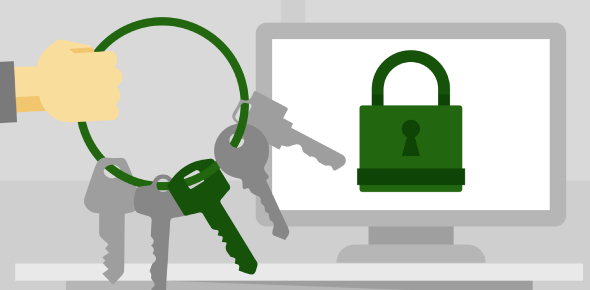# CompTIA Security+ Cryptography Quiz!

24 Questions | Attempts: 621Settings.

• 1.
Which of the following statements about the SHA (Security Hash Algorithm) are true?
• A.

• B.

• C.

It is used with encryption protocols

• D.

It is used with networking protocols

• 2.
Which of the following are encryption systems?
• A.

RC5

• B.

Blowfish

• C.

MAC

• D.

ARP

• 3.
Which of the following is true about Public/Private key pairs?
• A.

They form an essential part of Website security

• B.

They are used by Certificate security system

• C.

They are a pair of clear text passwords

• D.

They are obsolete.

• 4.
RSA is the encryption system used in cellular devices.
• A.

True

• B.

False

• 5.
What is the requirement for cryptography?
• A.

To avoid unauthorized access of information being stored

• B.

To avoid unauthorized access of network resources

• C.

To avoid unauthorized access of information being transmitted.

• D.

To avoid unauthorized access of network servers

• 6.
Which of the following is responsible for key issues relating to the security of inter-bank communications?
• A.

IETF

• B.

ISI

• C.

NSA

• D.

ABA

• 7.
Which of the following will be required to perform Cryptanalysis?
• A.

Mathematical tools

• B.

Analytical reasoning

• C.

Pattern finding

• D.

Mathematical reasoning

• E.

• 8.
When employing Caesar's Cipher key value of 3, which of the following will be the decrypted equivalent of JGOOQ?
• A.

HELLO

• B.

WHAT

• C.

WHEN

• D.

DATE

• 9.
Which of the following is an advantage of using conventional encryption?
• A.

It is the most secure

• B.

It is very fast

• C.

It is economical

• D.

None of the above

• 10.
The concept of public-key cryptography was introduced by Diffie-Hellman.
• A.

True

• B.

False

• 11.
Which of the following statements about Public Key Cryptography are true?
• A.

You need to have a security setup configured on both the sending as well as the receiving ends to implement Public Key Cryptography.

• B.

You do not need an existing security setup

• C.

Public key can only encrypt and private key can only decrypt.

• D.

Public key can encrypt as well as decrypt, private key can only decrypt

• E.

None of the above

• 12.
The keys are measured by bytes.
• A.

True

• B.

False

• 13.
A digital signature is synonymous to which of the following?
• A.

Finger print

• B.

Hand written signature

• C.

Blood sample

• D.

None of the above

• 14.
When employing message digest, if data does get modified, which of the following will be the result of that modification?
• A.

• B.

The resulting digest after data modification will contain a completely different value.

• C.

The messages will be dropped and retransmitted.

• D.

None of the above

• 15.
Which of the following are the two key-based algorithms?
• A.

Symmetric algorithm

• B.

Asymmetric algorithm

• C.

128-bit key algorithm

• D.

1024-bit key algorithm

• 16.
Which of the following can be termed as �brute force attack�?
• A.

Trying all combinations to break a code

• B.

Breaking into strong cryptography

• C.

Forcibly capturing all data being transmitted

• D.

None of the above

• 17.
Which of the following types of cryptography is possible?
• A.

Cryptography with keys

• B.

Cryptography without keys

• C.

Cryptography before encryption

• D.

Cryptography without encryption

• 18.
Cryptography without keys is more secure than cryptography with keys. T/F?
• A.

True

• B.

False

• 19.
Which of the following is required by the cipher when it is important to maintain a message as a secret?
• A.

Generate one cipher text for that message

• B.

Generate at least 5 cipher text for that message

• C.

Generate several cipher text.

• D.

None of the above.

• 20.
Which of the following is the most common attack faced by the DES algorithm?
• A.

DoS

• B.

Brute force attack

• C.

Code attack

• D.

None of the above

• 21.
Differential cryptanalysis is nothing but pattern studying.
• A.

True

• B.

False

• 22.
Which of the following is provided by IDEA?
• A.

High level security

• B.

Complex implementation

• C.

Ease of implementation

• D.

Average security level

• 23.
Which of the following best describes Ron�s Code 2?
• A.

64-bit block cipher

• B.

128-bit block cipher

• C.

64-bit stream cipher

• D.

128-bit stream cipher

• 24.
The block size in RC5 can range from 0 to 255. Y/N?
• A.

Yes

• B.

No

## Related TopicsBack to top
×

Wait!
Here's an interesting quiz for you.